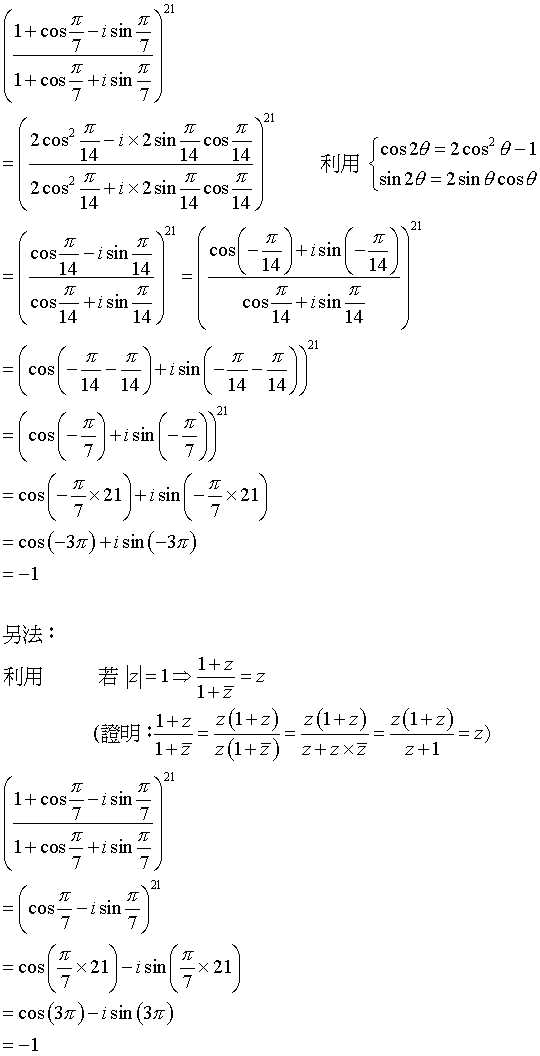# 例題：三角函數─隸美弗定理的運用

## 例題：三角函數─隸美弗定理的運用

$\left( {\frac{{1 + \cos \frac{\pi }{7} - i\sin \frac{\pi }{7}}}{{1 + \cos \frac{\pi }{7} + i\sin \frac{\pi }{7}}}} \right)^{21}.$TOP

﻿